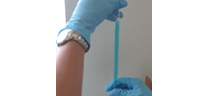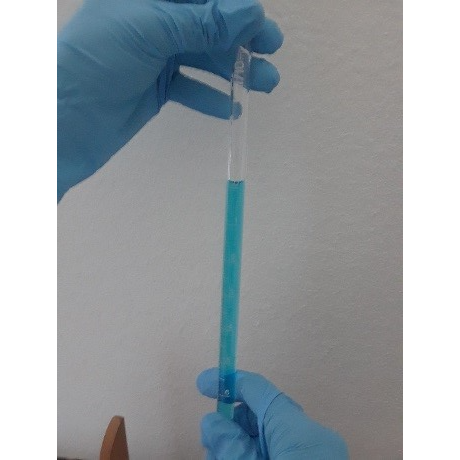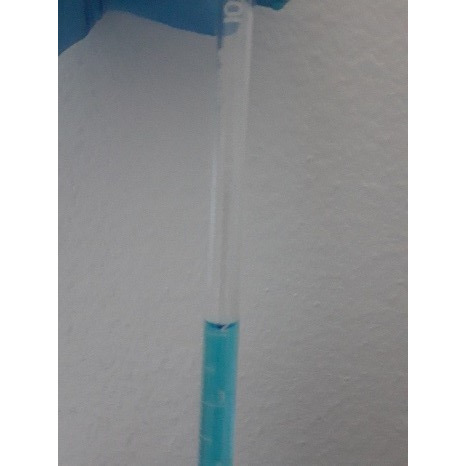# Experiment_612_Beer's Law_1_3_4

$$\newcommand{\vecs}{\overset { \rightharpoonup} {\mathbf{#1}} }$$ $$\newcommand{\vecd}{\overset{-\!-\!\rightharpoonup}{\vphantom{a}\smash {#1}}}$$$$\newcommand{\id}{\mathrm{id}}$$ $$\newcommand{\Span}{\mathrm{span}}$$ $$\newcommand{\kernel}{\mathrm{null}\,}$$ $$\newcommand{\range}{\mathrm{range}\,}$$ $$\newcommand{\RealPart}{\mathrm{Re}}$$ $$\newcommand{\ImaginaryPart}{\mathrm{Im}}$$ $$\newcommand{\Argument}{\mathrm{Arg}}$$ $$\newcommand{\norm}{\| #1 \|}$$ $$\newcommand{\inner}{\langle #1, #2 \rangle}$$ $$\newcommand{\Span}{\mathrm{span}}$$ $$\newcommand{\id}{\mathrm{id}}$$ $$\newcommand{\Span}{\mathrm{span}}$$ $$\newcommand{\kernel}{\mathrm{null}\,}$$ $$\newcommand{\range}{\mathrm{range}\,}$$ $$\newcommand{\RealPart}{\mathrm{Re}}$$ $$\newcommand{\ImaginaryPart}{\mathrm{Im}}$$ $$\newcommand{\Argument}{\mathrm{Arg}}$$ $$\newcommand{\norm}{\| #1 \|}$$ $$\newcommand{\inner}{\langle #1, #2 \rangle}$$ $$\newcommand{\Span}{\mathrm{span}}$$$$\newcommand{\AA}{\unicode[.8,0]{x212B}}$$

 Student Name Laboratory Date:  Date Report Submitted: ___________________________ Student ID Experiment Number and Title Experiment 612: Beer’s Law

Experiment 612:  Beer’s Law

Section 1:  Purpose and Summary

Determine the concentration of copper(II) ions in solution using spectrophotometric analysis.

Many substances absorb UV (ultraviolet) or visible light. When a substance absorbs certain frequencies (colors) of light, some of them are transmitted, and the solution appears colored. The color you see is the opposite of the color it absorbs in the basic color wheel (ROYGBV). For example, copper(II) sulfate solution is blue because the Cu2+ ion absorbs visible light in the 600 – 650 nm range (orange).

A spectrophotometer is an instrument used to measure the amount of light absorbed by a sample. A light source directs light into the sample and the amount of light absorbed is measured as either absorbance or % transmittance. If all the light passes through the sample without any absorption, then the absorbance reading on the spectrophotometer would be ‘zero’, and % transmittance is 100. The sample appears colorless.

In colored samples, the absorbance is related to the molar concentration of the absorbing substance in the solution. This relationship is known as Beer’s law and is given by the equation: A = abC, where A is the absorbance of the solution, a is the molar absorptivity of the substance, b is the path length of light passing through the solution, and C is the concentration of the solution in molarity (moles/L). If a and b are constant, then the absorbance, A, is directly proportional to the concentration, C. A solution of higher concentration absorbs more light, while a solution of lower concentration absorbs less light. If the absorbance of different solutions of known concentration is measured, a graph of absorbance vs. concentration results in a straight line.

In this experiment, students will prepare several dilute solutions from a standard stock solution. Using a spectrophotometer, students will measure the absorbance of each of the dilute solutions and construct a graph of absorbance vs concentration. Students will be given copper(II) sulfate of unknown concentration and measure its absorbance. Using this absorbance and the graph, students will determine the concentration of the unknown copper solution.

Section 2:  Safety Precautions and Waste Disposal

Safety Precautions:

Use of eye protection is recommended for all experimental procedures.

Spectrophotometers and cuvettes are expensive. Use them carefully.

Waste Disposal:

Copper(II) solutions should be disposed of in the Inorganic Hazardous Waste container.  Dissolved copper salts are very toxic to marine and aquatic life.

Section 3: Procedure

Part 1:  Preparation of dilute Cu2+ solutions

You will be preparing four dilute Cu2+ solutions from a 0.35 M standard Cu2+ solution.

 For this part of the experiment, gather the following glassware and materials.   Four (4) clean, and dry medium (150 mm) test tubes - labeled 1 through 4.   Two (2) 100-mL beakers – one labeled ‘water’ and the other labeled ‘Cu2+’  Large beaker – labeled ‘waste’  Two (2) 10-mL graduated pipets – one labeled ‘water’ and the other labeled ‘Cu2+’  Pipet bulb Rinse the beaker labeled ‘Cu2+’ with a small amount of the standard Cu2+ solution and dump the rinse into the ‘waste’ beaker. Fill this beaker, about half-full, of standard Cu2+ solution. Record the exact concentration of the standard Cu2+ solution on the data table below. Rinse the beaker labeled ‘water’ with a small amount of laboratory water and dump the rinse into the sink. Fill this beaker, about half-full, of laboratory water. Rinse each labeled graduated pipet three times with the substance/solution it will be used for. Each time dump the rinse into the ‘waste’ beaker. Be careful not to get any water or Cu2+ solution sucked into the pipet bulb. Now, you are ready to prepare the four dilute Cu2+ solutions. Each of the four test tubes will be filled with laboratory water and standard Cu2+ solutions with the amounts listed in the table below. Use your graduated pipets to withdraw the specified volumes. Make sure to record the actual volumes to two (2) decimal places. For example, if you must withdraw 2 mL of water, the water level can go beyond the 2 mL mark but record the exact volume, such as 2.01 mL.     To use the graduated pipet, first use the bulb to draw solution into the pipet above the zero mark.  Cap the end of the pipet with your finger.  Slowly allow air into the top of the pipet until the meniscus is just touching the ‘0.00’ calibration mark.  Move the end of the pipet over to your receiving test tube.  Drain the pipet until the meniscus is just touching the ‘2.00’ mark.  Remove the pipet and return the additional liquid back to the stock you have in your beaker.  2.00 mL has now been transferred.  Adjust these instructions for other volumes as appropriate.Cover and securely close each test tube with a small piece of parafilm. Shake each solution well.

 Test tube # Volume (mL) standard Cu2+ Volume (mL) laboratory water 1 2 8 2 4 6 3 6 4 4 8 2

Part 2. Absorbance Measurement

Follow instructions on the preparation and proper use of a spectrophotometer (Spectronic 20/200) (see Technique I: Use of Spectrophotometer of the laboratory manual for additional information). Turn on the spectrophotometer and allow it to warm up for 15 minutes. Set the wavelength to 620 nm. Absorbance measurements should all be done on the same day, using the same spectrophotometer.

 Using a wash bottle filled with laboratory water, rinse and then fill (about ¾ full) a cuvette with laboratory water. This is your ‘blank’ sample. Wipe the outside wall of cuvette with KimwipeTM and place it on the sample compartment in the spectrophotometer. Cover the sample compartment. Adjust spectrophotometer to ‘zero’ absorbance. Discard the laboratory water from the cuvette into the sink. You are now ready to measure the absorbance of the four dilute Cu2+ solutions. Rinse the cuvette three times with small portions of the dilute solutions you prepared in Part 2 of this experiment starting with test tube #1. Discard the rinse into the ‘waste’ beaker. Fill the cuvette with the solution (about ¾ full), wipe off the outside wall of the cuvette with KimwipeTM, place in the sample compartment, cover, and read the absorbance of the solution. Discard the solution in the waste beaker. Repeat steps 3 and 4 for all other dilute Cu2+ solutions. Record your data on the table below.

Concentration of standard Cu2+ solution (mol/L): __________________________________

 Test tube # Actual Volume (mL) of standard Cu2+ (V1) Actual Volume (mL) of laboratory water Total Volume (mL) of solution   (V2) Absorbance Concentration (M2)* 1 2 3 4

*see Section 4. Calculations

Part 3. Analysis of Unknown

 Obtain an unknown sample from your instructor. Record its identification code. Identification code for unknown: Repeat steps 1 – 3 of Part 2 above and record the absorbance of your unknown sample solution. Absorbance of unknown Cu2+ solution: Discard the solution into the ‘waste’ beaker.

Section 4: Calculations

SHOW YOUR WORK on the space provided below.

 Refer to a periodic table and calculate the molar mass of CuSO4×5H2O. Using this information, the mass of the solid, and the total volume of solution you made in Part 1, calculate the concentration (M) of the standard Cu2+ solution.    Molar mass of CuSO4×5H2O: ________________ g/mol    Show your equation here: Concentration of standard Cu2+ solution (M2):      ____________________ mol/L Using the dilution equation, M1V1 = M2V2, calculate the concentration of Cu2+ (M2) in each of the diluted solutions in Part 2. Record the values on the last column of table in Part 3.    Show one sample calculation here: After completing the table in Part 3, make a graph of absorbance (y-axis) vs. the concentration of Cu2+, M2 (x-axis). Use a graphing paper. Including a point at the origin (0,0), draw the best straight line among the points.  An example is included at the end of this procedure. Using the straight line on the graph you made, determine the concentration of Cu2+ in your unknown solution from its absorbance. Estimate the value from the graph. Concentration of Cu2+ in your unknown sample:    ____________________ mol/L Attach your graph to this lab report. Post-Lab Questions:    Why is it necessary to rinse the pipets with the solution to be used in them before using the pipets?            Why is it necessary to rinse out the cuvette with the solution to be used in it before making our measurements?              Why must we use the same cuvette for all measurements?              What is the purpose of “zeroing” the spectrophotometer using a “blank” solution (water)?              A 6.000 g sample mixture containing copper was dissolved in 100.0 mL solution. The absorbance of this solution was 0.477 at 620 nm. Use your Beer’s law graph to determine the concentration of copper ions in this solution. Then determine the mass percent copper in the sample mixture. Assume that the other components in the mixture did not interfere in the absorbance reading.

Example graph of data.  The solid data points are from the measurements.  The absorbance of an unknown solution is plotted on the graph with a hollow data point.  The concentration of the unknown can be determined graphically from the calibration line by drawing a vertical line to the x-axis.

Note:  This analysis may also be done by calculation.

Measured absorbance vs. Copper(II) Sulfate Concentration